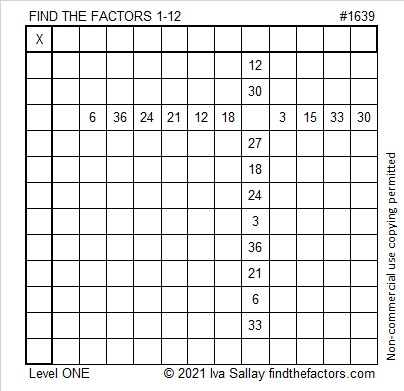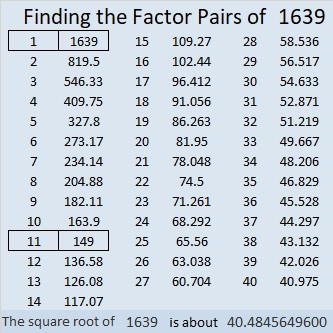# 1639 and Level 1

Contents

### Today’s Puzzle:

Write the numbers from 1 to 12 in both the first column and the top row so that those numbers and the given clues will make this puzzle function like a multiplication table.### Factors of 1639:

1 – 6 + 3 – 9 = -11 so 1639 is divisible by 11.

• 1639 is a composite number.
• Prime factorization: 1639 = 11 × 149.
• 1639 has no exponents greater than 1 in its prime factorization, so √1639 cannot be simplified.
• The exponents in the prime factorization are 1 and 1. Adding one to each exponent and multiplying we get (1 + 1)(1 + 1) = 2 × 2 = 4. Therefore 1639 has exactly 4 factors.
• The factors of 1639 are outlined with their factor pair partners in the graphic below.### More about the Number 1639:

1639 is the hypotenuse of a Pythagorean triple:
561 1540 1639, which is 11 times (51-140-149).

1639 is the 22nd nonagonal number because
22(7·22 – 5)/2 =
22(154 – 5)/2=
22(149)/2 =
11(149) = 1639.
Mathworld.Wolfram has illustrations of the first 5 nonagonal numbers.

This site uses Akismet to reduce spam. Learn how your comment data is processed.